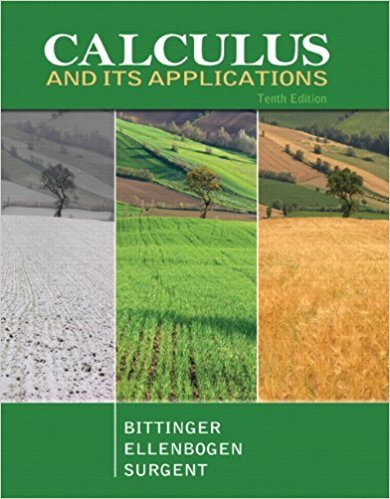×
×

# Solutions for Chapter 3.2: Logarithmic Functions## Full solutions for Calculus and Its Applications | 10th Edition

ISBN: 9780321694331Solutions for Chapter 3.2: Logarithmic Functions

Solutions for Chapter 3.2
4 5 0 388 Reviews
31
3
##### ISBN: 9780321694331

Calculus and Its Applications was written by and is associated to the ISBN: 9780321694331. This textbook survival guide was created for the textbook: Calculus and Its Applications, edition: 10. This expansive textbook survival guide covers the following chapters and their solutions. Chapter 3.2: Logarithmic Functions includes 122 full step-by-step solutions. Since 122 problems in chapter 3.2: Logarithmic Functions have been answered, more than 25049 students have viewed full step-by-step solutions from this chapter.

Key Calculus Terms and definitions covered in this textbook
• Axis of symmetry

See Line of symmetry.

• Branches

The two separate curves that make up a hyperbola

• Double-blind experiment

A blind experiment in which the researcher gathering data from the subjects is not told which subjects have received which treatment

• Expanded form of a series

A series written explicitly as a sum of terms (not in summation notation).

• Finite series

Sum of a finite number of terms.

• Half-plane

The graph of the linear inequality y ? ax + b, y > ax + b y ? ax + b, or y < ax + b.

• Law of sines

sin A a = sin B b = sin C c

See Polynomial function in x

• Leibniz notation

The notation dy/dx for the derivative of ƒ.

• Limit to growth

See Logistic growth function.

• Outliers

Data items more than 1.5 times the IQR below the first quartile or above the third quartile.

• Polar coordinate system

A coordinate system whose ordered pair is based on the directed distance from a central point (the pole) and the angle measured from a ray from the pole (the polar axis)

• Randomization

The principle of experimental design that makes it possible to use the laws of probability when making inferences.

• Reference triangle

For an angle ? in standard position, a reference triangle is a triangle formed by the terminal side of angle ?, the x-axis, and a perpendicular dropped from a point on the terminal side to the x-axis. The angle in a reference triangle at the origin is the reference angle

• Scalar

A real number.

• Solution of a system in two variables

An ordered pair of real numbers that satisfies all of the equations or inequalities in the system

• Sum of complex numbers

(a + bi) + (c + di) = (a + c) + (b + d)i

• Transitive property

If a = b and b = c , then a = c. Similar properties hold for the inequality symbols <, >, ?, ?.

• yz-plane

The points (0, y, z) in Cartesian space.

• z-coordinate

The directed distance from the xy-plane to a point in space, or the third number in an ordered triple.

×